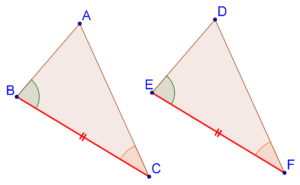# The ASA Criterion

Go back to  'Triangles-Quadrilaterals'

Consider the following two triangles, ∆ABC and ∆DEF:We are given that BC = EF, B = E and C = F. Can we say that the two triangles are congruent?

Let us first do a thought experiment and try to superimpose triangle DEF on triangle ABC. Align EF exactly with BC.

1. Since B = E, the direction of ED will be the same as the direction of BA.

2. Similarly, since C = F, the direction of FD will be the same as the direction of CA.

This means that the point of intersection of ED and FD (which is D) will coincide exactly with the point of intersection of BA and CA (which is A). Thus, since all the three vertices of the two triangles (can be made to) respectively coincide, the two triangles are congruent.

What we have described above is an intuitive proof of the ASA criterion. However, Mathematics requires rigor, and we must rigorously show the truth of the ASA criterion.